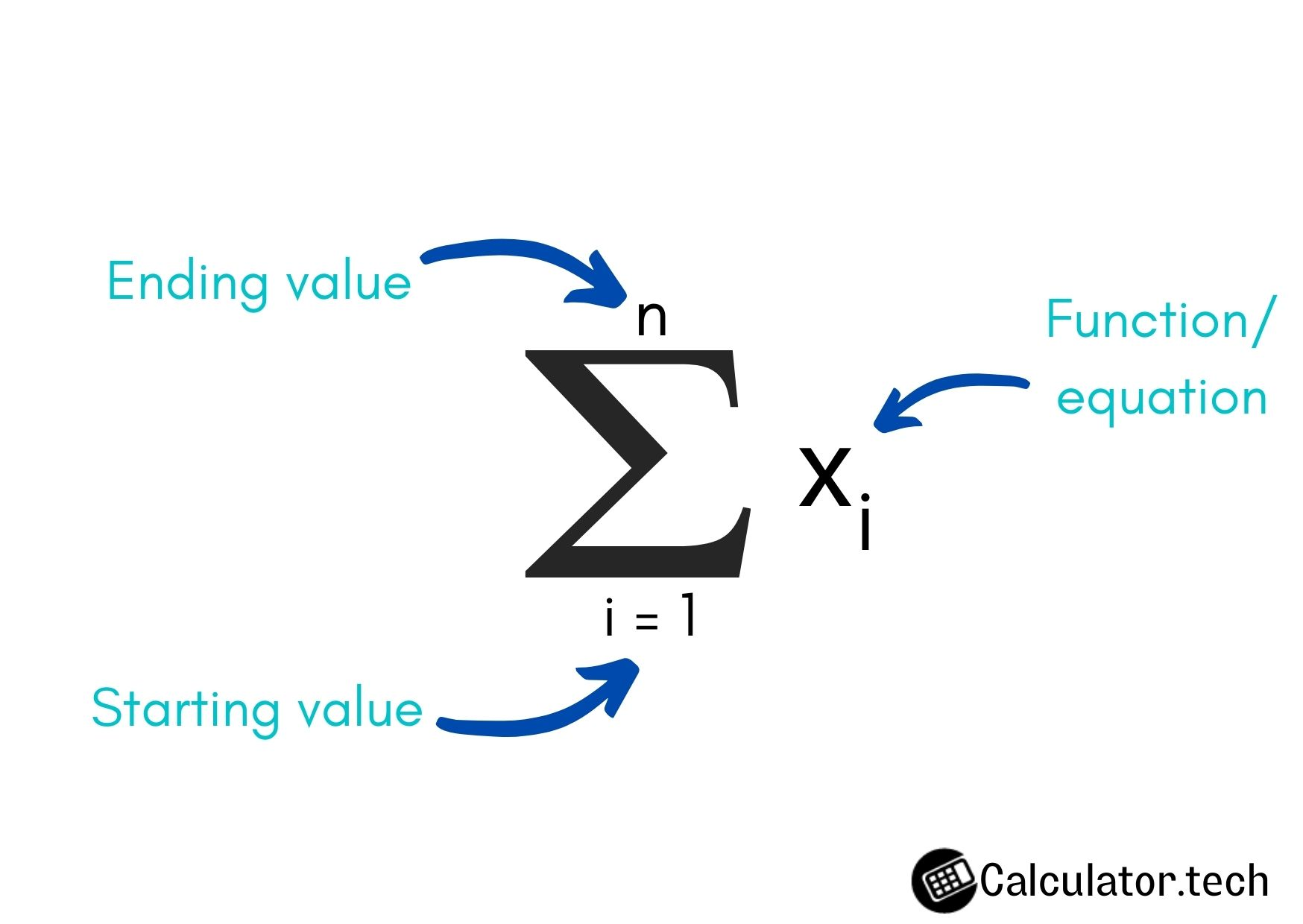# Summation Calculator

#### RESULTS

The summation calculator uses the sigma operator. It adds all the functions together. You can add separate numbers (series) or equations through this sigma notation calculator.

## How to use the summation solver?

You can calculate the sum of series or equations using the summation calculator.

1. Choose summation for series or equation according to need.
2. Choose the values you want to put at the place of the variable.
3. Enter "start" and "ending" values.
4. Enter the equation.

(For summing series enter the numbers separated by commas.)

Sigma sum calculator is also known as summation notation calculator as they both refer to the same thing.

## What is Summation?

Summation means “adding”. It is represented by sigma notation which isIn this picture, the letter “i” on the top of the summation notation is the first value to be put in the equation. The letter “n” represents the last value. While $$X_i$$ represents the equation.

## How to perform Summation?

Let’s learn to add or sum series and equations through examples.

### Example of "series" summation:

Solve;

$$\displaystyle\sum_{i=1}^5x_i$$

Step 1: Write the values separated by commas.

1, 2, 3, 4, 5

= 1 + 2 +3 + 4 +5

= 15

### Example of "equation" summation:

Solve;

$$\displaystyle\sum_{i=1}^5x^2$$

Step 1: Put the values in the equation one by one.

$$1^2 ,2^2 ,3^2 ,4^2 ,5^2$$

$$2 ,4 ,9 ,16 ,25$$

$$= 2 + 4 + 9 + 16 + 25$$

$$= 55$$

Other Languages
User Ratings
• Total Reviews 0
• Overall Rating 0/5
• Stars
Reviews
No Review Yet